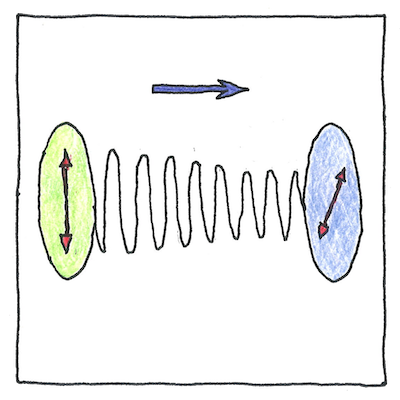# 1845-1913 Magneto-optic and electro-optic effects

Tom Sharp

## Magneto-optic and electro-optic effects

• Magneto-optic and electro-optic effects
• named after the scientists who discovered them:
• the electro-optic Kerr effect, 1875
• the magneto-optic Kerr effect, 1877
• the Cotton effect, 1895
• the Voigt effect, 1898
• the Zeeman effect, 1902
• the Cotton-Mouton effect, 1907
• and the Stark-Lo Surdo effect, 1913.
• A magnetic force rotates a polarized light.
• A translucent material exhibits birefringence under an electric field.
• A magnetized surface rotates the light reflected from it.
• Proximity to the absorption band of a material affects optical rotation.
• A gas exhibits birefringence in a transverse magnetic field.
• A magnetic field splits a spectral line into multiple parts.
• A liquid exhibits birefringence in a transverse magnetic field.
• An electric field splits a spectral line into multiple parts.

• Light and electromagnetism are related,
• which Michael Faraday was the first to show
• by shining a polarized light through lead glass
• and applying a magnetic force in the direction of the light
• so that the polarization rotated
• in proportion to the strength of magnetic force.

## Electro-optic Kerr effect

• Apply a voltage
• to a translucent material.
• A light shining through it
• shows birefringence,
• where the light
• oscillating in the direction
• of the electric field
• and the light ocsillating
• across the electric field
• are refracted at different angles.

## Magneto-optic Kerr effect

• Light reflected from a magnetized surface
• is rotated and reflected with different intensities
• according to the direction of magnetization
• so the crystallization of a metallic surface
• can be made visible using a polarized light.

## Cotton effect

• The plane of polarization of light
• shining through an optically active liquid
• rotates in one direction at higher frequencies
• close to the absorption band of the liquid
• and in the opposite direction at lower frequencies.

## Voigt effect

• An optically active gas
• exhibits a double refraction of light
• proportional to the square
• of a transverse magnetic field.
• You may investigate further
• for how to calculate
• eigenvalues and eigenvectors
• from dielectric cubic tensors.

## Zeeman effect

• A magnetic field
• splits spectral lines
• into multiple parts
• and their distance
• depends on the strength
• of the field,
• which lets us map
• magnetic fields
• of stars
• and gives birds
• their magnetic
• compass.

## Cotton-Mouton effect

• A liquid exhibits
• birefringence
• in a transverse
• magnetic field.
• It’s like
• the Voigt effect
• but with a liquid
• It’s like
• the Kerr effect
• but with an magnetic field
• instead of an electric field.

## Stark-Lo Surdo effect

• The Stark-Lo Surdo effect
• is like the Zeeman effect
• but spectral lines split
• in an electric field.

## Thus

• light and electromagnetic forces
• Thus the magneto optical drive
• that can write data using a magnetic field
• and read it using polarized light.
• Thus high-speed photography
• using a Kerr cell to modulate light
• by changing an electric field.
• Thus the Kerr microscope
• that visualizes magnetic domains
• of a metal surface.
• Thus ellipsometry
• for visualizing dielectric properties
• of thin films.
• Thus polarimetry
• for remote sensing
• from outer space.
• Thus atomic line filters
• for lidar mapping
• through clouds and jungles.
• Thus using
• a Zeeman slower
• for laser cooling.
• Thus electroabsorption/emission spectroscopy
• for analyzing physiochemical
• properties of a sample.

## Limited senses

• Dung beetles roll their dung balls in straight lines
• guided by the polarization of the sky, which they see.
• Polarized sunglasses can show us the same information
• though we don’t need to roll dung balls in straight lines.

Faraday proposed in 1847 that light was a high-frequency electromagnetic vibration.

Just a word about polarization of light. Light is a wave, but the waving has two arms perpendicular to each other and perpendicular to the direction of propagation. A polarized light eliminates one of these arms, so that the light waves in only one direction.